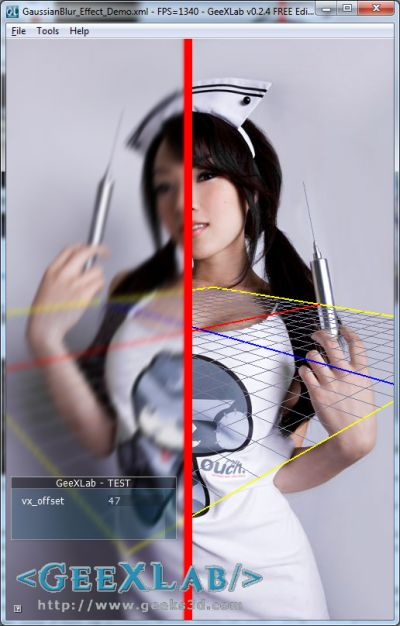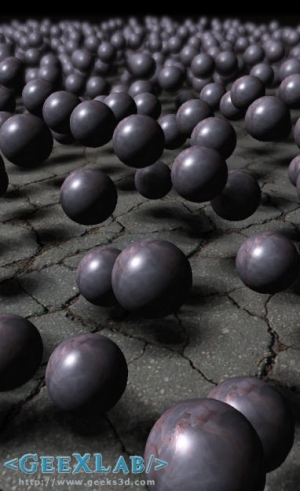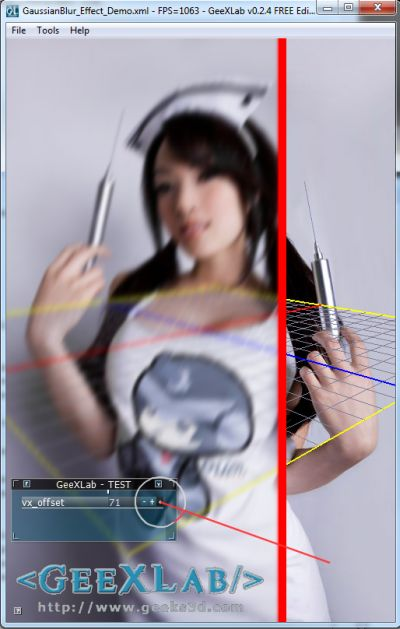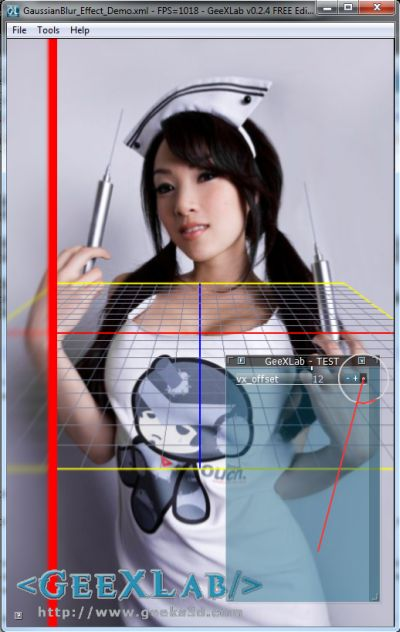# (Shader Library) Gaussian Blur Post Processing Filter in GLSLA cute nurse half blurred in GeeXLab

Gaussian blur is a filter widely used in computer graphics. In real time 3D, Gaussian blur is used in many effects like depth of field or bloom.Depth of field effect uses Gaussian blur filter

Many years ago, I wrote a tutorial about image filtering with GLSL where I gave an example of Gaussian filter. Few days ago, Daniel Rakos wrote a detailed article about an efficient Gaussian blur with linear sampling.

Since I’m always looking for new effects and shaders for GeeXlab, I decided to implement Daniel’s work in a GeeXLab demo.

Basically, the gaussian blur filter works with two passes: one vertical and the other horizontal. Why two passes? Wikipedia gives us the answer:

A Gaussian blur effect is typically generated by convolving an image with a kernel of Gaussian values. In practice, it is best to take advantage of the Gaussian Blur’s linearly separable property by dividing the process into two passes. In the first pass, a one-dimensional kernel is used to blur the image in only the horizontal or vertical direction. In the second pass, another one-dimensional kernel is used to blur in the remaining direction. The resulting effect is the same as convolving with a two-dimensional kernel in a single pass, but requires fewer calculations.

Here is the complete vertical gaussian filter used in the demo (you can download the demo at the end of the post):

```[Vertex_Shader]
void main(void)
{
gl_Position = ftransform();
gl_TexCoord = gl_MultiTexCoord0;
}
uniform sampler2D sceneTex; // 0

uniform float rt_w; // render target width
uniform float rt_h; // render target height
uniform float vx_offset;

float offset = float[]( 0.0, 1.3846153846, 3.2307692308 );
float weight = float[]( 0.2270270270, 0.3162162162, 0.0702702703 );

void main()
{
vec3 tc = vec3(1.0, 0.0, 0.0);
if (gl_TexCoord.x<(vx_offset-0.01))
{
vec2 uv = gl_TexCoord.xy;
tc = texture2D(sceneTex, uv).rgb * weight;
for (int i=1; i<3; i++)
{
tc += texture2D(sceneTex, uv + vec2(0.0, offset[i])/rt_h).rgb \
* weight[i];
tc += texture2D(sceneTex, uv - vec2(0.0, offset[i])/rt_h).rgb \
* weight[i];
}
}
else if (gl_TexCoord.x>=(vx_offset+0.01))
{
tc = texture2D(sceneTex, gl_TexCoord.xy).rgb;
}
gl_FragColor = vec4(tc, 1.0);
}
```

And here is the complete horizontal gaussian filter used in the demo:

```[Vertex_Shader]
void main(void)
{
gl_Position = ftransform();
gl_TexCoord = gl_MultiTexCoord0;
}
uniform sampler2D sceneTex; // 0

uniform float rt_w; // render target width
uniform float rt_h; // render target height
uniform float vx_offset;

float offset = float[]( 0.0, 1.3846153846, 3.2307692308 );
float weight = float[]( 0.2270270270, 0.3162162162, 0.0702702703 );

void main()
{
vec3 tc = vec3(1.0, 0.0, 0.0);
if (gl_TexCoord.x<(vx_offset-0.01))
{
vec2 uv = gl_TexCoord.xy;
tc = texture2D(sceneTex, uv).rgb * weight;
for (int i=1; i<3; i++)
{
tc += texture2D(sceneTex, uv + vec2(offset[i])/rt_w, 0.0).rgb \
* weight[i];
tc += texture2D(sceneTex, uv - vec2(offset[i])/rt_w, 0.0).rgb \
* weight[i];
}
}
else if (gl_TexCoord.x>=(vx_offset+0.01))
{
tc = texture2D(sceneTex, gl_TexCoord.xy).rgb;
}
gl_FragColor = vec4(tc, 1.0);
}
```

The vx_offset uniform allows to control the X position of the vertical red line:vx_offset = 0.71vx_offset = 0.12

The vx_offset is controled by the tweak bar and the value is divided by 100: 71 gives 0.71.

And what about the background image???
Just visit this page: KusoPOP Nurse by *VampBeauty or this one: vampbeauty.com 😉

With that kind of image, post FX coding is a real pleasure…

### *** You need GeeXLab 0.2.4 or higher to run this demo. ***

Unzip the demo somewhere, start GeeXLab and drop in the main window the demo source code (GaussianBlur_Effect_Demo.xml). Gaussian blur shaders are localized in the PostFX_GaussianBlur_Lib.xml file. The shortcut [Ctrl+R] in GeeXLab allows to reload the demo once you have modified the source code.

Enjoy!

## 11 thoughts on “(Shader Library) Gaussian Blur Post Processing Filter in GLSL”

1.Korvin77

*looks at shots* ummm such a nice advertising posters. I like this tool now 😀 we all must have it )))

2.JeGX Post Author

Thanks for the separable convolution link. The explanation is simple and clear.

3.Daniel Rákos

Nice post and nice image 😀

I’m glad to see that my work inspired you to write the demo and article!

4.Eric Haines

Nice to have this in the collection! Since your post, Daniel’s fixed his code’s weights a bit so that they sum to one. I’d recommend fixing your weights to match his.

5.JeGX Post Author

Thanks Eric, updated!

6.Gelko

ulala that girl is HaWT, any hd pic of that? hehe

7.Florian Märkl

You could make tc a vec4 and change all the
“texture2D(sceneTex, ).rgb” to
“texture2D(sceneTex, )” and
“gl_FragColor = vec4(tc, 1.0);”
to
“gl_FragColor = vec4(tc);”
and the alpha value is blended as well!

8.memmeck asem

nice boobs 😮

9.Crixe

I see a picture of Linda Le(Vampy bit Me) there. Nice.